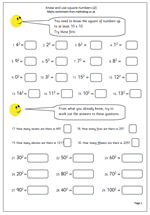# Year 6 Maths worksheet: Square numbers (2)Here we have a follow up page for the year 6 maths worksheet on square numbers published on Thursday. Further practice at remembering square numbers and using this knowledge to work out square numbers of multiples of 10.

Know and use square numbers (2)